## Abstract

This article presents the effects of nonlinear thermal radiation and induced magnetic field on viscoelastic fluid flow toward a stagnation point. It is assumed that there exists a kind of chemical reaction between chemical species A and B. The diffusion coefficients of the two chemical species in the viscoelastic fluid flow are unequal. Since chemical species B is a catalyst at the horizontal surface, hence homogeneous and heterogeneous schemes are of the isothermal cubic autocatalytic reaction and first order reaction respectively. The transformed governing equations are solved numerically using Runge–Kutta integration scheme along with Newton’s method. Good agreement is obtained between present and published numerical results for a limiting case. The influence of some pertinent parameters on skin friction coefficient, local heat transfer rate, together with velocity, induced magnetic field, temperature, and concentration profiles is illustrated graphically and discussed. Based on all of these assumptions, results indicate that the effects of induced magnetic and viscoelastic parameters on velocity, transverse velocity and velocity of induced magnetic field are almost the same but opposite in nature. The strength of heterogeneous reaction parameter is very helpful to reduce the concentration of bulk fluid and increase the concentration of catalyst at the surface.

## Keywords

Induced magnetic field; Homogeneous–heterogeneous reactions; Nonlinear thermal radiation; Viscoelastic fluid

## Nomenclature

u- velocity component in x direction

v- velocity component in y direction

${\textstyle B_{1}{\mbox{,}}B_{2}}$- magnetic components in x and y directions

${\textstyle B_{o}}$- magnetic component in free stream flow

${\textstyle C_{f}}$- skin friction coefficient

${\textstyle C_{p}}$- specific heat at constant pressure

x- distance along the surface

y- distance normal to the surface

f- dimensionless velocity

${\textstyle N_{u}}$- local Nusselt number

${\textstyle P_{r}}$- Prandtl number

${\textstyle q_{w}}$- heat transfer

T- dimensional fluid temperature

${\textstyle u_{w}}$- stretching velocity at the wall

${\textstyle u_{e}}$- stretching velocity at the free stream

${\textstyle T_{w}}$- wall temperature

${\textstyle T_{\infty }}$- ambient temperature

${\textstyle R_{ex}}$- local Reynolds number

${\textstyle g{\mbox{,}}g^{'}}$- dimensionless induced magnetic velocities field in y and x directions respectively

${\textstyle a{\mbox{,}}c}$- stretching rate at the wall and free stream

${\textstyle K{\mbox{,}}K_{s}}$- strength of Homogeneous and Heterogeneous reaction parameters

${\textstyle G(\eta )}$- concentration of Specie A

${\textstyle G^{'}(\eta )}$- conc. gradient of specie A

${\textstyle H(\eta )}$- conc. of cpecie B

${\textstyle H^{'}(\eta )}$- conc. gradient of specie B

### Greek symbols

λ- stretching ratio parameter

Γ- viscoelastic parameter

δ- ratio of diffusion coefficients chemical specie A to B

ε- reciprocal magnetic Prandtl number

κ- thermal conductivity

η- similarity variable

θ- dimensionless temperature

ϑ- kinematics viscosity

α- thermal diffusivity

σ- electrical conductivity

${\textstyle {\sigma }^{_{\ast }}}$- Stefan–Boltzmann constant

${\textstyle k^{_{\ast }}}$- mean absorption coefficient

β- magnetic parameter

σ- electric conductivity

θ- dimensionless temperature

ψ- stream function

${\textstyle {\mu }_{e}}$- magnetic permeability

## 1. Introduction

The study of fluid flow over a horizontal stretchable surface has extensive applications in pharmaceutical companies (i.e. medicinal pharmaceutical technology), production of polythene and paper, polymer extrusion, cooling of elastic sheets, fiber technology, production of plastic materials, science and engineering technology. It is worth mentioning that almost all the fluids mentioned above are materials (fluids) which exhibit viscous and elastic characteristics when undergoing deformation. Viscoelastic fluid flow over a stretching surface was investigated by Rajagopal et al. . Chethan et al.  examined the slip condition on viscoelastic liquid flow over an exponentially stretching surface. Rashidi et al.  analyzed the effects of Soret and Dufour on magnetohydrodynamic viscoelastic fluid flow past a vertical stretching surface. Singh and Agarwal  studied the variable thermal conductivity effect on the flow of viscoelastic fluid over a permeable exponentially stretching surface. Hayat et al.  discussed the boundary layer analysis of viscoelastic nanofluid flow over a stretching cylinder. A stagnation point is a point in a flow field where the local velocity is zero. In fluid dynamics, when this occurs at the surface of an object, the fluid flow is often brought to rest. To induce such fluid to flow, Paul Richard Heinrich Blasius in 1908 considered the report of Prandtl Ludwig together with the principle of stretching fluid layers at the free stream . It is pertinent to note that in the work of Blasius, the fluid motion is maintained by the stretching of fluid layers at the free stream. Thereafter, Hiemenz  presented two dimensional stagnation-point flow over a flat plate. Abolbashari et al.  presented the analysis of non-Newtonian fluid flow induced by stretching sheet. Heat transfer characteristics of viscoelastic fluid past a stretching surface in the presence of thermal radiation and viscous dissipation was presented by Cortell . The dynamics of micropolar fluid at constant vortex viscosity toward a stagnation point formed on a melting stretchable surface can be seen in ${\textstyle K{\underset {\cdot }{o}}r{\acute {\imath }}k{\underset {\cdot }{o}}}$. Just of recent, Sandeep et al.  discussed the dual solutions of governing equation which models Stagnation-point flow of a Jeffrey nanofluid over a stretching surface with induced magnetic field and chemical reaction.

A chemical reaction has occurred when two or more reactants yield product. If the product of a chemical reaction is a catalyst which even speeds up the chemical reaction, then such chemical reaction is said to have passed through autocatalysis. A chain of autocatalytic reactions was first proposed by Lotka . Sapre  investigated the problem of simultaneous chemical reaction and diffusion in the presence of catalyst. Williams et al.  reported that this kind of reaction can occur between bulk of fluid and surface of a plate in which the boundary layer is formed upon. Merkin  adopted an order of magnitude (scaling analysis) together with the same assumptions of large Reynolds number on Navier–Stoke’s equation as reported by Ludwig Prandtl; and then presented a simple model for the interaction between homogeneous (in the bulk of fluid) and heterogeneous (at the surface) reaction involving two chemical species A and B within a thin layer formed on a surface in fluid flow. Explanation on the case of unequal diffusion coefficients of chemical species A and B can be found in Chaudhary and Merkin . Mabood et al.  analyzed the flow of chemically reacting fluid over a stretching sheet. Boundary layer analysis of MHD Casson fluid flow over a permeable stretching sheet in the presence of chemical reaction was depicted by Gireesha et al. . Animasaun  considered the case of nth order of chemical reaction in Casson fluid flow with temperature dependent plastic dynamic viscosity. Khan and Pop  investigated a case of homogeneous–heterogeneous reaction which occurs in viscoelastic fluid flow. A homogeneous–heterogeneous reaction on Powell–Eyring fluid flow over a stretching sheet was analytically discussed by Hayat et al. . Bachok et al.  studied the homogeneous–heterogeneous reactions on stagnation-point flow past a stretching surface.

Magnetohydrodynamics (MHD) is the science of motion of electrically conducting fluid in the presence of magnetic field. It was deduced that on 3rd of October 1942, a scientist/Engineer called H. Alfven reported that if a conducting liquid is placed in a constant magnetic field, motion of the liquid may give rise to a force called electromotive force (e.m.f.) which produces electric currents. A kind of electromotive force which induces current and also tends to generate what is called Lorentz force; further reported that owing to the magnetic field, these currents give mechanical forces which change the state of motion of the liquid . Ever since then, Engineers and researchers have reported its application in fusion reactors, dispersion of metals, metallurgy, design of MHD pumps, MHD generator and MHD flow meters, etc. Liron and Wilhelm  stated that MHD boundary layer flow of a viscous and electrically conducting fluid in the presence of a transverse magnetic field is observed in various technical systems employing liquid metal and plasma flow. Sandeep and Sulochana  illustrated an unsteady micropolar fluid flow over a stretching/shrinking surface in the presence of magnetic field. The heat transfer analysis of Casson fluid flow past a permeable exponentially stretching surface with magnetic field was discussed by Raju et al. . Micropolar fluid flow over a linear stretching surface with magnetic field was analytically studied by Siddheshwar and Mahabaleshwar . Magnetic Reynolds number is an important parameter in the study of effects of induced magnetic field on fluid flow. Rashidi et al.  solved a system of nonlinear differential equations modeling the flow of a Newtonian magnetic lubricant squeeze film with magnetic induction and reported the effect of magnetic Reynolds number. Transient magnetohydrodynamics free convection flow of four different types of water based nanofluid past a vertical surface is presented by Freidoonimehr et al. .

Motsa and Animasaun  considered a case in which the order of magnitude of diffusion-thermo (Dufour effect) is more significant in boundary layer fluid flow in the presence of Lorentz force at initial unsteady stage and final steady stage. Sheikholeslami and Rashidi  adopted Control Volume-based Finite Element Method to simulate nanofluid flow in the presence of a non-uniform magnetic field. The aim of the study was extended in  and it was concluded that increasing magnetic number leads to augmentation of the Nusselt number. In most cases of electrically conducting fluid flow in the presence of magnetic field, the magnetic Reynolds number of the flow may not be realistic to be assumed small in magnitude; hence, the induced magnetic field is not negligible. Ali et al.  examined the induced magnetic effect on stagnation-point flow toward a stretching surface and concluded that the induced magnetic parameter develops the momentum boundary layer thickness. An induced magnetic field effect on stagnation point flow of a nanofluid past a stretching surface was considered by Pal and Mandal . In all the literatures mentioned above, it is worth noticing that little attention has been given to investigate the motion of viscoelastic fluid toward a stretching sheet in the presence of nonlinear thermal radiation. In addition, no attempt has been made to investigate the case of unequal diffusivities of homogeneous–heterogeneous reaction which occurs in the viscoelastic fluid as it flows toward a stagnation point and thereafter. The nonlinear governing equations that model the fluid flow are considered in this study and non-dimensionalized by using suitable similarity variables. The transformed governing equations are solved numerically via Runge–Kutta along with Newton’s method. The influence of selected dimensionless parameters on velocity, induced magnetic field, temperature and concentration profiles along with the friction factor and local Nusselt number is illustrated graphically and presented in tabular form.

## 2. Mathematical analysis

Consider a steady, electrically conducting viscoelastic fluid flow toward a stagnation point and thereafter along a horizontal stretchable surface in the presence of induced magnetic field and homogeneous–heterogeneous reactions.

The physical model along with the coordinate system is shown in Fig. 1. The stretching sheet is considered along the ${\textstyle x}$-axis and ${\textstyle y}$-axis is normal to it. The magnetic Reynolds number of the viscoelastic flow is not small in magnitude; hence, the induced magnetic field is highly significant. It is assumed that the applied magnetic field is of uniform strength ${\textstyle B_{o}}$. It is also assumed that an induced magnetic field is applied in ${\textstyle y}$-direction and the parallel component ${\textstyle B_{1}}$ approaches the value ${\textstyle B_{e}=B_{o}}$ in the free stream flow. In addition, normal component of the induced magnetic field ${\textstyle B_{2}}$ vanishes near the wall. The temperatures near and far away from the stretching sheet are denoted as ${\textstyle T_{w}}$ and ${\textstyle T_{\infty }}$ respectively. The stretching and free streams velocities are ${\textstyle u_{w}=cx}$ and ${\textstyle u_{e}=ax}$ respectively, where c and a are positive constants which represent stretching rate. It is assumed that there exists a homogeneous–heterogeneous reaction between chemical species A and B as the viscoelastic fluid flows toward a stagnation point and thereafter. This kind of chemical reaction is referred to as autocatalytic since chemical specie B is known as catalyst. Following Merkin , it is assumed that within the boundary layer we have isothermal cubic autocatalytic reaction given by

 $A+2B\quad \Rightarrow \quad 3B{\mbox{,}}\quad rate=k_{c}{ab}^{2}{\mbox{.}}$
(1)

while on the catalyst surface we have the single, isothermal first order chemical reaction of the form

 $A\quad \Rightarrow \quad B{\mbox{,}}\quad rate=k_{s}a{\mbox{.}}$
(2)

where a and b are the concentrations of the chemical species A and B   respectively. Here, ${\textstyle k_{c}}$ and ${\textstyle k_{s}}$ are known as the reaction rate coefficients which may not be actually referred to as a constant because it includes all the likely parameters that may affect reaction rate except concentration which we have explicitly accounted for in Eqs. (1) and (2). Considering all the assumptions stated above and following the mathematical formulations given by Merkin , Chaudhary and Merkin , Nazar et al.  and Ishak et al. , the governing boundary layer equations for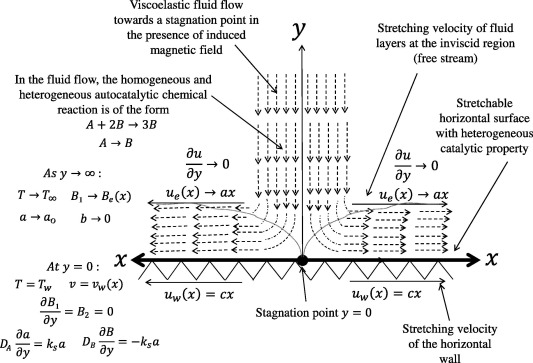Figure 1. Physical configuration and coordinate system.

Continuity equation

 ${\frac {\partial u}{\partial x}}+{\frac {\partial v}{\partial y}}=$$0{\mbox{,}}$
(3)

Momentum equation

 $u{\frac {\partial u}{\partial x}}+v{\frac {\partial u}{\partial y}}=$$u_{e}{\frac {\partial u_{e}}{\partial x}}-{\frac {\mu }{4\pi \rho }}B_{e}{\frac {\partial B_{e}}{\partial x}}+$${\frac {\mu }{\rho }}{\frac {{\partial }^{2}u}{\partial y^{2}}}+$${\frac {\mu }{4\pi \rho }}\left(B_{1}{\frac {\partial B_{1}}{\partial x}}+\right.$$\left.B_{2}{\frac {\partial B_{1}}{\partial y}}-B_{e}{\frac {\partial B_{e}}{\partial x}}\right)-$${\frac {k_{o}}{\rho }}\left(u{\frac {{\partial }^{3}u}{\partial x\partial y^{2}}}+\right.$$\left.{\frac {\partial u}{\partial y}}{\frac {{\partial }^{2}v}{\partial y^{2}}}+\right.$$\left.v{\frac {{\partial }^{3}u}{\partial y^{3}}}\right){\mbox{,}}$
(4)

Induced magnetic field equation

 ${\frac {\partial B_{1}}{\partial x}}+{\frac {\partial B_{2}}{\partial y}}=$$0{\mbox{,}}$
(5)

 $u{\frac {\partial B_{1}}{\partial x}}+v{\frac {\partial B_{1}}{\partial y}}=$$B_{1}{\frac {\partial u}{\partial x}}+B_{2}{\frac {\partial u}{\partial y}}+$${\mu }_{e}{\frac {{\partial }^{2}B_{1}}{\partial y^{2}}}{\mbox{,}}$
(6)

Energy equation

 $u{\frac {\partial T}{\partial x}}+v{\frac {\partial T}{\partial y}}=$${\frac {\kappa }{\rho c_{p}}}{\frac {{\partial }^{2}T}{\partial y^{2}}}-$${\frac {1}{\rho c_{p}}}{\frac {\partial q_{r}}{\partial y}}{\mbox{,}}$
(7)

Homogeneous–heterogeneous reaction model for the concentrations of reactants A and B, as stated in the reaction scheme Eqs. (1) and (2)

 $u{\frac {\partial a}{\partial x}}+v{\frac {\partial a}{\partial y}}=$$D_{A}{\frac {{\partial }^{2}a}{\partial y^{2}}}-K_{c}{ab}^{2}{\mbox{,}}$
(8)

 $u{\frac {\partial b}{\partial x}}+v{\frac {\partial b}{\partial y}}=$$D_{B}{\frac {{\partial }^{2}b}{\partial y^{2}}}+K_{c}{ab}^{2}{\mbox{.}}$
(9)

Eqs. (3), (4), (5), (6), (7), (8) and (9) are subject to boundary conditions

 $u=u_{w}(x){\mbox{,}}\quad v=v_{w}(x){\mbox{,}}\quad {\frac {\partial B_{1}}{\partial y}}=$$B_{2}=0{\mbox{,}}\quad T=T_{w}{\mbox{,}}\quad D_{A}{\frac {\partial a}{\partial y}}=$$k_{s}a{\mbox{,}}\quad D_{B}{\frac {\partial b}{\partial y}}=$$-k_{s}a\quad at\quad y=0$
(10)

 $u\quad \rightarrow \quad u_{e}(x){\mbox{,}}\quad {\frac {\partial u}{\partial y}}\quad \rightarrow \quad 0{\mbox{,}}\quad B_{1}\quad \rightarrow \quad B_{e}(=$$B_{o}x){\mbox{,}}\quad T\quad \rightarrow \quad T_{\infty }{\mbox{,}}\quad a\quad \rightarrow \quad a_{o}{\mbox{,}}\quad b\quad \rightarrow \quad 0\quad as\quad y\quad \rightarrow \quad \infty$
(11)

The magnetic diffusivity of the fluid ${\textstyle {\mu }_{e}}$ is equivalent to ${\textstyle {\frac {1}{4\pi \sigma }}}$. To convert the governing equations into set of nonlinear ordinary differential equations, we now introduce the following similarity transformation:

 $u=cx{\frac {df}{d\eta }}{\mbox{,}}\quad v=-{\sqrt {\vartheta c}}f(\eta ){\mbox{,}}\quad \eta =$$y{\sqrt {\frac {c}{\vartheta }}}{\mbox{,}}\quad G(\eta )=$${\frac {a}{a_{o}}}{\mbox{,}}\quad H(\eta )={\frac {b}{a_{o}}}{\mbox{,}}$

 $B_{1}=B_{o}x{\frac {dg}{d\eta }}{\mbox{,}}\quad B_{2}=-B_{o}{\sqrt {\frac {c}{\vartheta }}}g(\eta ){\mbox{,}}\quad \theta (\eta )=$${\frac {T-T_{w}}{T_{w}-T_{\infty }}}{\mbox{,}}$
(12)

 $q_{r}=-{\frac {4{\sigma }^{_{\ast }}}{3k^{_{\ast }}}}{\frac {\partial T^{4}}{\partial y}}=$$-{\frac {4{\sigma }^{_{\ast }}}{3k^{_{\ast }}}}{\frac {\partial }{\partial y}}(T\times T\times T\times T)$
(13)

where ${\textstyle {\sigma }^{_{\ast }}}$ and ${\textstyle k^{_{\ast }}}$ are the Stefan–Boltzman constant and the mean absorption coefficient respectively. In this study, it is assumed that the temperature differences within the flow are not sufficiently small. In view of this, it may not be realistic to simplify the radiative heat flux by expanding ${\textstyle T^{4}}$ in a Taylor series about ${\textstyle T_{\infty }}$ and then neglecting higher order terms. Implicit differentiation may be adopted to simplify Eq. (13) and then substitute into energy Eq. (7)

 $u{\frac {\partial T}{\partial x}}+v{\frac {\partial T}{\partial y}}=$$\alpha {\frac {{\partial }^{2}T}{\partial y^{2}}}+{\frac {4{\sigma }^{_{\ast }}}{3k^{_{\ast }}\rho c_{p}}}4T^{3}{\frac {{\partial }^{2}T}{\partial y^{2}}}{\mbox{,}}$
(14)

If we are to consider ${\textstyle {\theta }_{w}={\frac {T_{w}}{T_{\infty }}}}$, the similarity variable related to energy equation is equivalent to

 $T=T_{\infty }[1+({\theta }_{w}-1)\theta ]$

The new form is adopted to parameterize the nonlinear term of thermal radiation in the energy equation. It is pertinent to remark that the variables defined in Eq. (12) automatically satisfy Eqs. (3) and (5). Upon substituting Eq. (12) into (3), (4), (5), (6), (7), (8), (9), (10) and (11), the following nonlinear coupled ordinary differential equations are obtained

 ${\frac {d^{3}f}{d{\eta }^{3}}}-\left({\frac {df}{d\eta }}{\frac {df}{d\eta }}-\right.$$\left.f{\frac {d^{2}f}{d{\eta }^{2}}}\right)+M\left({\frac {dg}{d\eta }}{\frac {dg}{d\eta }}-\right.$$\left.g{\frac {d^{2}g}{d{\eta }^{2}}}-1\right)+{\lambda }^{2}+$$\Gamma \left(f{\frac {d^{4}f}{d{\eta }^{4}}}-{\frac {df}{d\eta }}{\frac {d^{3}f}{d{\eta }^{3}}}+\right.$$\left.{\frac {d^{2}f}{d{\eta }^{2}}}{\frac {d^{2}f}{d{\eta }^{2}}}\right)=$$0{\mbox{,}}$
(15)

 $\epsilon {\frac {d^{3}g}{d{\eta }^{3}}}+f{\frac {d^{2}g}{d{\eta }^{2}}}-$$g{\frac {d^{2}f}{d{\eta }^{2}}}=0{\mbox{,}}$
(16)

 $\left(1+{\frac {1}{R}}{\left[1+({\theta }_{w}-1)\theta \right]}^{3}\right){\frac {d^{2}\theta }{d{\eta }^{2}}}+$$\left(3{\frac {1}{R}}({\theta }_{w}-1){\left[1+({\theta }_{w}-1)\theta \right]}^{2}\right){\frac {d\theta }{d\eta }}{\frac {d\theta }{d\eta }}+$$P_{r}f{\frac {d\theta }{d\eta }}=0{\mbox{,}}$
(17)

 ${\frac {d^{2}G}{d{\eta }^{2}}}+S_{cA}\left(f{\frac {dG}{d\eta }}-\right.$$\left.{KGH}^{2}\right)=0{\mbox{,}}$
(18)

 $\delta {\frac {d^{2}H}{d{\eta }^{2}}}+S_{cB}\left(f{\frac {dH}{d\eta }}+\right.$$\left.{KGH}^{2}\right)=0{\mbox{.}}$
(19)

The case of homogeneous–heterogeneous chemical reaction in which the diffusion coefficients of chemical species A and B are not of the same magnitude (unequal diffusivities) is considered in this study; hence, it is valid to retain dimensionless governing Eqs. (18) and (19). The dimensionless boundary conditions are now of the form

 $f=S{\mbox{,}}\quad {\frac {df}{d\eta }}=1{\mbox{,}}\quad g=$$0{\mbox{,}}\quad {\frac {d^{2}g}{d{\eta }^{2}}}=0{\mbox{,}}\quad \theta =$$1{\mbox{,}}\quad {\frac {dG}{d\eta }}=K_{s}G{\mbox{,}}\quad \delta {\frac {dH}{d\eta }}=$$-K_{s}G\quad at\quad \eta =0{\mbox{.}}$
(20)

 ${\frac {df}{d\eta }}\quad \rightarrow \quad \lambda {\mbox{,}}\quad {\frac {d^{2}f}{d{\eta }^{2}}}\quad \rightarrow \quad 0{\mbox{,}}\quad {\frac {dg}{d\eta }}\quad \rightarrow \quad 1{\mbox{,}}\quad \theta \quad \rightarrow \quad 0{\mbox{,}}\quad G\quad \rightarrow \quad 1{\mbox{,}}\quad H\quad \rightarrow \quad 0\quad as\quad \eta \quad \rightarrow \quad \infty {\mbox{.}}$
(21)

where Γ is the viscoelastic parameter, M is the magnetic parameter, ε   is the reciprocal magnetic Prandtl number, ${\textstyle P_{r}}$ is the Prandtl number, λ   is the velocity ratio parameter, ${\textstyle S_{cA}}$ is the Schmidt number for chemical specie, ${\textstyle A{\mbox{,}}S_{cB}}$ is the Schmidt number for chemical specie, ${\textstyle B{\mbox{,}}K}$ is known as the strength of homogeneous reaction, ${\textstyle K_{s}}$ is the strength of heterogeneous reaction, S   is the suction parameter and ${\textstyle \delta =D_{A}/D_{B}}$ is the ratio of diffusion coefficient, which are represented below.

 $M={\frac {\mu H_{o}^{2}}{4\pi \rho c^{2}}}{\mbox{,}}\quad \epsilon =$${\frac {1}{4\pi \sigma \vartheta }}{\mbox{,}}\quad \Gamma =$${\frac {{cK}_{o}}{\vartheta \rho }}{\mbox{,}}\quad R={\frac {3\kappa k^{_{\ast }}}{16{\sigma }^{_{\ast }}T_{\infty }^{3}}}{\mbox{,}}\quad P_{r}=$${\frac {\vartheta }{\alpha }}{\mbox{,}}\quad \lambda ={\frac {a^{2}}{c^{2}}}{\mbox{,}}\quad \quad S_{cA}=$${\frac {\vartheta }{D_{A}}}{\mbox{,}}\quad S_{cB}={\frac {\vartheta }{D_{B}}}$
 $K={\frac {k_{c}a_{o}^{2}}{c}}{\mbox{,}}\quad K_{s}={\frac {k_{s}}{D_{A}{\frac {c}{\vartheta }}}}{\mbox{,}}\quad S=$${\frac {v_{w}}{\sqrt {vc}}}$
(22)

Physical quantities of engineering interest are the shear stress coefficient ${\textstyle c_{f}}$ (friction factor) and the local Nusselt number ${\textstyle {Nu}_{x}}$ are given by

 $c_{f}={\frac {{\tau }_{w}}{\rho U_{w}^{2}}}{\mbox{,}}\quad {Nu}_{x}=$${\frac {{xq}_{w}}{k(T_{w}-T_{\infty })}}$
(23)

Here, ${\textstyle {\tau }_{w}}$ is the wall shear stress and ${\textstyle q_{w}}$ is the wall heat flux, which are given by

 ${\tau }_{w}=\mu {\left({\frac {\partial u}{\partial y}}\right)}_{y=0}{\mbox{,}}\quad \quad q_{w}=$$-k{\left({\frac {\partial T}{\partial y}}\right)}_{y=0}$
(24)

Using the variables in Eq. (12) we obtain

 ${Re}_{x}^{1/2}c_{f}=f^{''}(0){\mbox{,}}\quad \quad {Re}_{x}^{-1/2}Nu=$$-{\theta }^{'}(0)$
(25)

## 3. Numerical procedure

The nonlinear ordinary differential equations Eqs. (15), (16), (17), (18) and (19) subject to boundary conditions Eqs. (20) and (21) are solved numerically using Runge–Kutta and Newton’s method given by Mallikarjuna et al. . We assumed the unspecified initial conditions for unknown variables, and the transformed first order differential equations are integrated numerically as an initial valued problem to a given terminal point. We can check the accuracy of the assumed missing initial condition, by comparing the calculated value of the different variables at the terminal point with the given value by the existence of the difference in improved values such that the missing initial conditions must be obtained. The calculations are carried out by a program in MATLAB package.

## 4. Results and discussion

The influence of some pertinent parameters on velocity, induced magnetic field, temperature and concentration profiles along with the friction factor coefficient and local Nusselt number is discussed and presented through graphs and tables. Table 1 depicts the validation of the present results with the already published literature. Table 1 shows that the values of ${\textstyle f^{''}(0)}$ and ${\textstyle -\theta (0)}$ with variation in M are in favorable agreement with previously published data in the literature by Ali et al. . This proves the validity of the present results along with the accuracy of the numerical technique we used in this study.

Table 1. Comparison of the skin friction coefficient and local Nusselt number with variation in M   when ${\textstyle \lambda =3{\mbox{,}}\Gamma =0{\mbox{,}}R=0{\mbox{,}}{\theta }_{w}=}$$0{\mbox{,}}P_{r}=0.72{\mbox{,}}S_{cA}=0{\mbox{,}}S_{cB}=0{\mbox{,}}K=$$0$ and ${\textstyle K_{s}=0}$.
M ${\textstyle C_{f}}$ Ali et al.  ${\textstyle N_{ux}}$ Ali et al.  ${\textstyle C_{f}}$ Present ${\textstyle N_{ux}}$ Present
0.1 4.70928 0.97902 4.70927 0.97902
0.5 4.62764 0.97617 4.62765 0.97617
1 4.52158 0.97240 4.52158 0.97240

The effect of magnetic field parameter on longitudinal velocity ${\textstyle f^{'}(\eta )}$, transverse velocity of induced magnetic field ${\textstyle g(\eta )}$ and induced magnetic field ${\textstyle g^{'}(\eta )}$ profiles of the flow is plotted in Figure 2, Figure 3 and Figure 4 respectively. It can be deduced that an increase in magnetic field parameter (M  ) depreciates both velocity ${\textstyle f^{'}(\eta )}$ and velocity of induced magnetic ${\textstyle g^{'}(\eta )}$ profiles. The decrease we observed in all these figures can be traced to the presence of lorentz force. As magnitude of parameter M   increases, the lorentz force dominates and tends to increase the viscous forces. This effect consequently leads to decrease in the longitudinal velocity of transverse velocity of inducing magnetic field ${\textstyle g(\eta )}$ as shown in Fig. 3. A significant decrease in transverse velocity of induced magnetic field with M   is highly significant at the free stream. The behavior of ${\textstyle g^{''}(\eta )}$ with an increase in M is illustrated in Fig. 5. This profile decreases near the wall (${\textstyle 0\leqslant \eta \leqslant 0.99}$) only. Table 2 further unravels the effects of increasing the magnitude of M   on ${\textstyle f^{''}(0)}$. It is observed that ${\textstyle f^{''}(0)}$ decreases with M.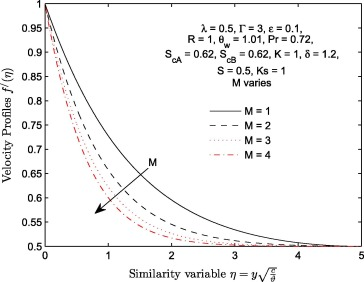Figure 2. Velocity profiles ${\textstyle f^{'}(\eta )}$. For different values of magnetic field parameter M.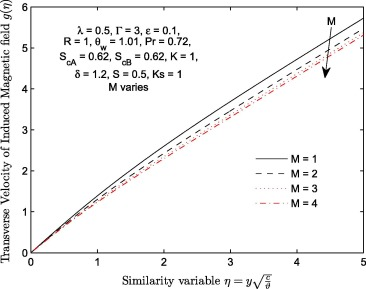Figure 3. Transverse velocity profiles ${\textstyle g(\eta )}$ for different values of magnetic field parameter M.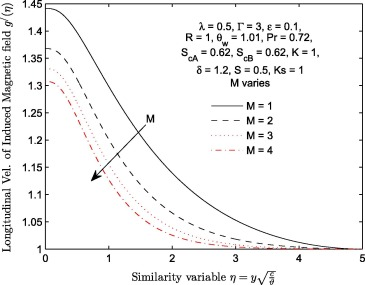Figure 4. Longitudinal velocity profiles ${\textstyle g^{'}(\eta )}$ for different values of magnetic field parameter M on.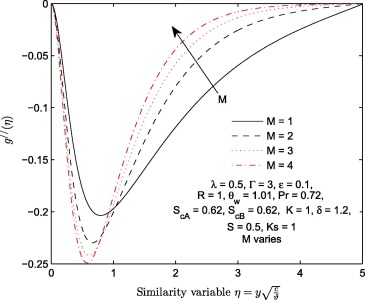Figure 5. Variation in the magnitude of magnetic field parameter M   with ${\textstyle g^{''}(\eta )}$.

Table 2. Numerical values of ${\textstyle f^{''}(0)}$ and ${\textstyle f^{'''}(0)}$ with variations in Γ and M   when ${\textstyle \lambda =0.8{\mbox{,}}\epsilon =0.1{\mbox{,}}R=1{\mbox{,}}{\theta }_{w}=}$$1.01{\mbox{,}}P_{r}=0.72{\mbox{,}}S_{cA}=0.62{\mbox{,}}S_{cB}=$$0.62{\mbox{,}}K=1{\mbox{,}}\delta =1.2{\mbox{,}}S=0.5$ and ${\textstyle K_{s}=1}$.
${\textstyle M=\Downarrow }$ ${\textstyle \Gamma =3}$ ${\textstyle \Gamma =\Downarrow }$ ${\textstyle M=3}$
${\textstyle f^{''}(0)}$ ${\textstyle f^{'''}(0)}$ ${\textstyle g^{''}(0)}$ ${\textstyle f^{''}(0)}$ ${\textstyle f^{'''}(0)}$ ${\textstyle g^{''}(0)}$
1 ${\textstyle -0.076563}$ 0.002769 1.155076 1 ${\textstyle -0.315704}$ 0.419162 1.093213
2 ${\textstyle -0.147728}$ 0.088140 1.131197 2 ${\textstyle -0.227888}$ 0.218492 1.109898
3 ${\textstyle -0.191399}$ 0.156035 1.118931 3 ${\textstyle -0.191399}$ 0.156035 1.118931

At a constant magnitude of lorentz force (${\textstyle M=1}$), the effect of viscoelastic parameter (Γ  ) on longitudinal velocity ${\textstyle f^{'}(\eta )}$, transverse velocity of induced magnetic field ${\textstyle g(\eta )}$ and induced magnetic field ${\textstyle g^{'}(\eta )}$ profiles of the flow is plotted in Figure 6, Figure 7 and Figure 8 respectively. It is shown that an increase in viscoelastic parameter (Γ  ) leads to an increase in both velocity ${\textstyle f^{'}(\eta )}$ and velocity of induced magnetic ${\textstyle g^{'}(\eta )}$ profiles. The increase we noticed in all these figures can be traced to the reduction in the strength of viscous force. As we all known, fluid with lower viscous forces holding their molecules flow faster than the fluid with high viscous forces. This effect consequently leads to increase in the longitudinal velocity of transverse velocity of induced magnetic field ${\textstyle g(\eta )}$ as shown in Fig. 7. The behavior of ${\textstyle g^{''}(\eta )}$ with an increase in the magnitude of Γ is illustrated in Fig. 9. This profile increases near the wall (${\textstyle 0\leqslant \eta \leqslant 0.99}$) only. Table 2 further explains the effects of increasing the magnitude of Γ   on ${\textstyle f^{''}(0)}$. It is observed that ${\textstyle f^{''}(0)}$ increases with Γ.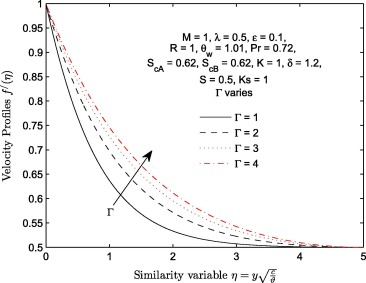Figure 6. Velocity profiles ${\textstyle f^{'}(\eta )}$ for different values of viscoelastic parameter Γ.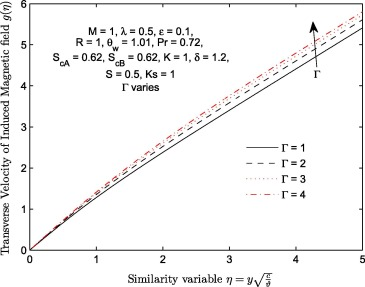Figure 7. Transverse velocity profiles ${\textstyle g(\eta )}$ for different values of viscoelastic parameter Γ.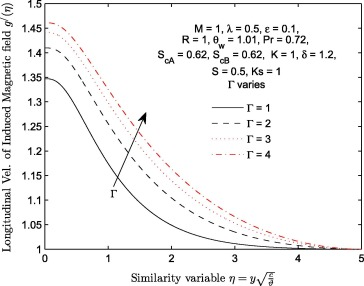Figure 8. Longitudinal velocity profiles ${\textstyle g^{'}(\eta )}$ for different values of viscoelastic parameter Γ.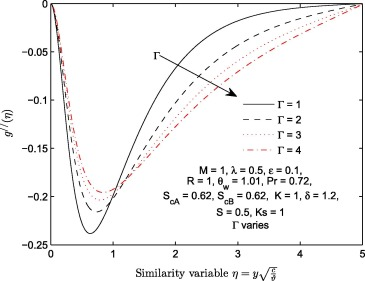Figure 9. Variation in the magnitude of viscoelastic parameter Γ   with ${\textstyle g^{''}(\eta )}$.

The effects of velocity stretching ratio parameter (λ  ) on ${\textstyle f(\eta ){\mbox{,}}f^{'}(\eta ){\mbox{,}}g^{'}(\eta ){\mbox{,}}\theta (\eta ){\mbox{,}}G(\eta )}$ and ${\textstyle H(\eta )}$ are shown in Figure 10, Figure 11, Figure 12, Figure 13, Figure 14 and Figure 15. Transverse velocity profile ${\textstyle f(\eta )}$ increases significantly with λ   few distance away from the wall. The longitudinal velocity ${\textstyle f^{'}(\eta )}$ profiles increase negligibly near the wall (${\textstyle 0\leqslant \eta \leqslant 0.09}$) and significantly near the free stream (${\textstyle \eta >1.85}$). Velocity of induced magnetic field ${\textstyle g^{'}(\eta )}$ decreases with an increase in velocity stretching ratio parameter (λ  ) near the wall (${\textstyle 0\leqslant \eta \leqslant 1.03}$) and increases negligibly thereafter. The temperature function ${\textstyle \theta (\eta )}$ decreases with λ. Figure 14 and Figure 15 display the effect of λ on concentration of specie A and specie B   when ratio of momentum diffusivity to mass diffusivity ${\textstyle S_{cA}=S_{cB}=0.62}$, strengths of homogeneous and heterogeneous chemical reactions ${\textstyle K=K_{s}=1}$. It is observed that ${\textstyle G(\eta )}$ increases with λ   while ${\textstyle H(\eta )}$ decreases with the same parameter. It is further deduced that increase in the magnitude of λ   has negligible effect on ${\textstyle H(\eta =0)}$ and significant effect on ${\textstyle G(\eta =0)}$.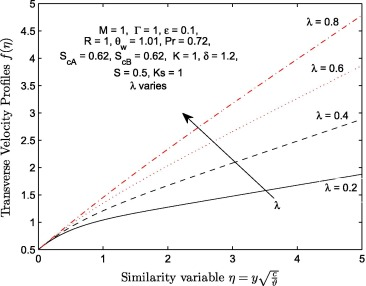Figure 10. Transverse velocity profiles ${\textstyle f(\eta )}$ for different values of velocity ratio parameter λ.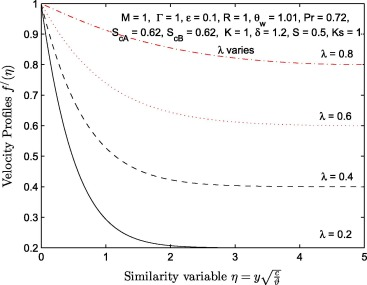Figure 11. Velocity profiles ${\textstyle f^{'}(\eta )}$ for different values of velocity ratio parameter λ.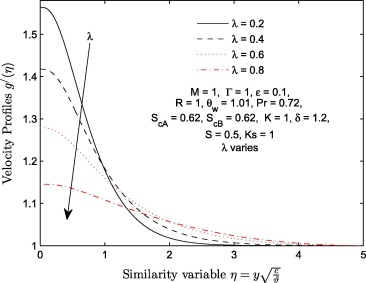Figure 12. Velocity profiles ${\textstyle g^{'}(\eta )}$ for different values of velocity ratio parameter λ.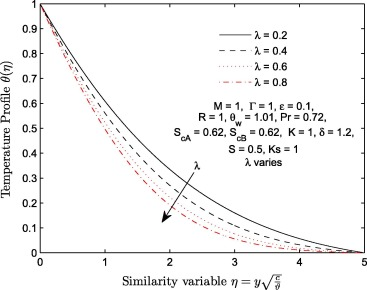Figure 13. Temperature profiles ${\textstyle \theta (\eta )}$ for different values of velocity ratio parameter λ.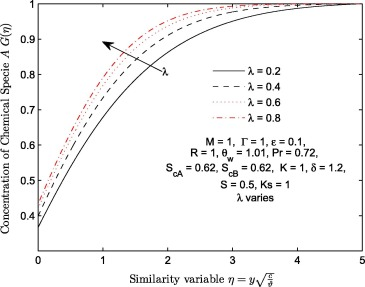Figure 14. Effects of velocity ratio parameter λ on concentration of chemical specie A  ${\textstyle G(\eta )}$.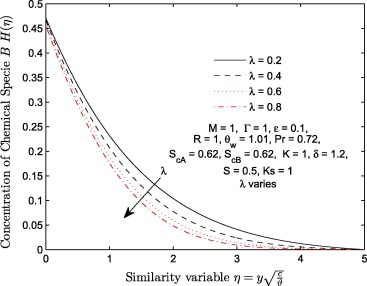Figure 15. Effects of velocity ratio parameter λ on concentration of chemical specie B   (catalyst) ${\textstyle H(\eta )}$.

Effects of thermal radiation R   and temperature parameter ${\textstyle {\theta }_{w}}$ on temperature profiles are illustrated in Figure 16 and Figure 17 respectively. At a constant value of ${\textstyle {\theta }_{w}=1.01}$, temperature profile ${\textstyle \theta (\eta )}$ decreases with an increase in the magnitude of R. The effect of radiation parameter R is to reduce the temperature significantly in the flow region. Thermal radiation is found to be energy transfer by the emission of electromagnetic waves which carry heat energy away as the fluid flows. Since the increase in radiation parameter means increase in the rate at which heat energy is released from the flow region, this accounts for decrease in temperature distribution as the magnitude of parameter R increases. It is very important to remark that this effect actually depends on the definition of parameter R  ; here, it is defined as the ratio of ${\textstyle 3\kappa k^{_{\ast }}}$ to ${\textstyle 16{\sigma }^{_{\ast }}T_{\infty }^{3}}$. In most published articles, if thermal radiation parameter is defined otherwise, increase in the parameter leads to increase in temperature. Certainly, this is not realistic (i.e. can temperature still increase when energy is transferred out of the fluid domain?). This may depend on the physical model. For more details on this, see  and . Also, Fig. 17 depicts an increase in ${\textstyle \theta (\eta )}$ with ${\textstyle {\theta }_{w}}$. The increase we observed in ${\textstyle \theta (\eta )}$ with ${\textstyle {\theta }_{w}}$ reduces as ${\textstyle {\theta }_{w}}$ grows large.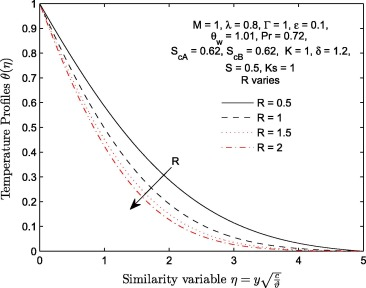Figure 16. Temperature profiles ${\textstyle \theta (\eta )}$ for different values of radiation parameter R.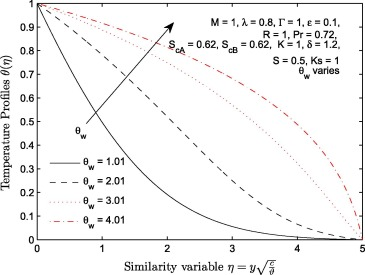Figure 17. Temperature profiles ${\textstyle \theta (\eta )}$ for different values of temperature parameter R.

The behavior of concentration of chemical specie A and chemical specie B with an increase in the strength of homogeneous chemical reaction K   and strength of heterogeneous chemical reaction ${\textstyle K_{s}}$ is illustrated in Figure 18 and Figure 19. It is noticed that the boundary conditions at the wall and at the free stream are satisfied. The concentration of chemical specie A  ${\textstyle G(\eta )}$ decreases negligibly with K. This decreasing effect is only near the wall. It is observed that the concentration of chemical specie B   (i.e. catalyst) ${\textstyle G(\eta )}$ decreases significantly near the wall. This significantly decreasing effect can be traced to the amount of magnitude assigned to parameter δ. This accounts for the reason why δ has no significant effect on the concentration of chemical specie A  ${\textstyle G(\eta )}$ since magnitude of K   and ${\textstyle K_{s}}$ is fixed; see Fig. 20 for more details. As shown in Fig. 19, higher values of the strength of heterogeneous reaction ${\textstyle K_{s}}$ result in the increment of ${\textstyle G(\eta )}$ and ${\textstyle H(\eta )}$. It is worth mentioning that at all points of η   within ${\textstyle \left[0{\mbox{,}}1.95\right]}$, the increase in the magnitude of ${\textstyle K_{s}}$ from 1 to 3 results in a significant increase of ${\textstyle G(\eta )}$ and ${\textstyle H(\eta )}$ profiles. This significant increase of ${\textstyle G(\eta )}$ and ${\textstyle H(\eta )}$ reduces as ${\textstyle K_{s}}$ grows larger. At a fixed value of ${\textstyle K_{s}}$, it is observed that the coefficients of ${\textstyle G^{'}(\eta )}$ decrease with K; see Table 3. Also, it is observed in Table 3 that the coefficients of ${\textstyle H^{'}(\eta )}$ increase more significantly with ${\textstyle K_{s}}$ when ${\textstyle K=1}$.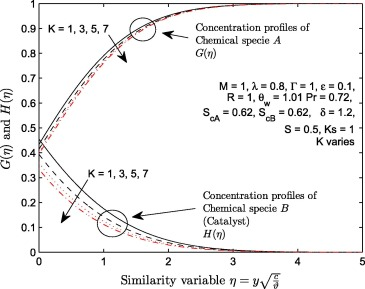Figure 18. Variation in the strength of homogeneous parameter (K  ) with ${\textstyle G(\eta )}$ and ${\textstyle H(\eta )}$.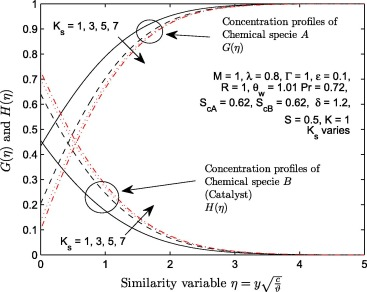Figure 19. Variation in the strength of heterogeneous parameter (${\textstyle K_{s}}$) with ${\textstyle G(\eta )}$ and ${\textstyle H(\eta )}$.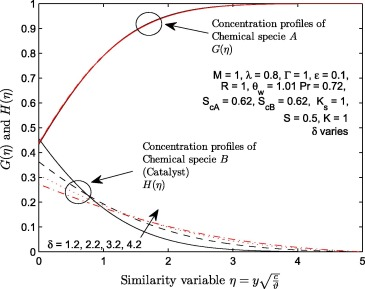Figure 20. Variation in the ratio of diffusion coefficient parameter (δ  ) with ${\textstyle G(\eta )}$ and ${\textstyle H(\eta )}$.

Table 3.

Numerical values of ${\textstyle G^{'}(0)}$ and ${\textstyle H^{'}(0)}$ with variations in K   and ${\textstyle K_{s}}$ when ${\textstyle \lambda =0.8{\mbox{,}}\epsilon =0.1{\mbox{,}}R=1{\mbox{,}}{\theta }_{w}=}$$1.01{\mbox{,}}P_{r}=0.72{\mbox{,}}S_{cA}=0.62{\mbox{,}}S_{cB}=$$0.62{\mbox{,}}\Gamma =1{\mbox{,}}\delta =1.2{\mbox{,}}S=$$0.5$ and ${\textstyle M=1}$.

${\textstyle K=\Downarrow }$ ${\textstyle K_{s}=1}$ ${\textstyle K_{s}=\Downarrow }$ ${\textstyle K=1}$
${\textstyle G^{'}(0)}$ ${\textstyle H^{'}(0)}$ ${\textstyle G^{'}(0)}$ ${\textstyle H^{'}(0)}$
1 0.43296989 ${\textstyle -0.36080824}$ 1 0.43296989 ${\textstyle -0.36080824}$
2 0.55214357 ${\textstyle -0.46011964}$ 2 0.42197286 ${\textstyle -0.35164405}$
3 0.41414673 ${\textstyle -0.34512228}$ 3 0.60884911 ${\textstyle -0.50737425}$

## 5. Conclusions

This study deals with the induced magnetic field effect on viscoelastic fluid flow past a stretching sheet in the presence of nonlinear thermal radiation and homogeneous–heterogeneous reactions. The arising sets of governing nonlinear coupled partial differential equations are transformed into a set of nonlinear coupled ordinary differential equations using self-similarity transformations, which are then solved numerically. The conclusions are as follows:

• the nonlinear thermal radiation improves the temperature profiles and suppresses the rate of heat transfer. At fixed value of R  , decrease in temperature distribution from the wall to the free stream is minimum when magnitude of ${\textstyle {\theta }_{w}}$ is small compared to maximum pattern of decreasing when magnitude of ${\textstyle {\theta }_{w}}$ is large.
• variation in the magnitude of ratio of diffusion coefficient is an increasing function of concentration of the catalyst only within the fluid domain.
• stretching ratio parameter and viscoelastic parameter have tendency to enhance the heat transfer rate. The effects of increasing magnitude of Γ   on ${\textstyle f^{'}(\eta ){\mbox{,}}g(\eta ){\mbox{,}}g^{'}(\eta )}$ and ${\textstyle g^{''}(\eta )}$ are the opposite of the effects of increasing M on the same set of profiles.
• an increase in the magnetic field parameter depreciates the friction factor coefficient and heat transfer rate.
• strength of heterogeneous reaction parameter is very helpful to reduce the concentration of bulk fluid ${\textstyle G(\eta )}$ and enlarge the concentration of the catalyst ${\textstyle H(\eta )}$ at the surface.
• at a constant value of Γ, transverse velocity profile is an increasing function of velocity ratio λ.

### Document informationPublished on 12/04/17

Licence: Other

### Document Score0

Views 20
Recommendations 0